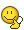BrainDen.com - Brain Teasers
• 0

# Order in a random set of alphabet letters

## Question

Suppose you wrote all the letters to the alphabet down in a random sequence. What is the probability that you write down at least 5 letters in proper alphabetical order?

For clarity (hopefully): for a letter to be in proper alphabetical order, the letter does not have to be moved or switched to the left past another letter in the given list. When deciding how many letters in a given random list are in order note that Letters need not be in adjacent order A,B,C,D,...) to be in proper alphabetical order. See examples below for more clarity.

Examples:

A L C E H. .... 4 letters are in proper alphabetical order (by dropping L)

ZYXWU ..... 1 letter is in proper letter order, pick one

HDAZV ........ 2 letters are in proper letter order, AZ for example

## Recommended Posts

• 0

Let pi be the probability that exactly i letters are ordered.
The answer is 1 - Sumi=1,4 (pi).##### Share on other sites

• 0

Let

pi be the probability that exactly i letters are ordered.

The answer is 1 - Sumi=1,4 (pi).Lol
##### Share on other sites

• 0

OK so I guess that approach is not helpful then ...I love puzzles of this type because when you see the solution it usually leads to ....

##### Share on other sites

• 0

This problem reminds me of another unsolvable BMAD puzzle involving the task of sorting DVDs using insertion sort.I think back then, Yoruichi-San, pointed us to a Wikipedia article that showed how to calculate an upper bound.

##### Share on other sites

• 0

This problem reminds me of another unsolvable BMAD puzzle involving the task of sorting DVDs using insertion sort.I think back then, Yoruichi-San, pointed us to a Wikipedia article that showed how to calculate an upper bound.

Here it is: https://en.m.wikipedia.org/wiki/Erdős–Szekeres_theorem

Surprisingly, I think this might hold the key to this question.

The theorem says that, for any given r and s, any sequence of at least (r-1)(s-1)+1 orderable elements has an ascending subsequence of length r or a descending subsequence of length s. In our case, we have 26 orderable elements, which suggests that every string contains an ascending or descending subsequence of length 6. Symmetry tells us that the probability of just an ascending subsequence of length 6 is at least 50%.

Edited by gavinksong
##### Share on other sites

• 0

This problem reminds me of another unsolvable BMAD puzzle involving the task of sorting DVDs using insertion sort.I think back then, Yoruichi-San, pointed us to a Wikipedia article that showed how to calculate an upper bound.

Here it is: https://en.m.wikipedia.org/wiki/Erdős–Szekeres_theorem

Surprisingly, I think this might hold the key to this question.

The theorem says that, for any given r and s, any sequence of at least (r-1)(s-1)+1 orderable elements has an ascending subsequence of length r or a descending subsequence of length s. In our case, we have 26 orderable elements, which suggests that every string contains an ascending or descending subsequence of length 6. Symmetry tells us that the probability of just an ascending subsequence of length 6 is at least 50%.

You could be right in that the theorem holds a key, but I am unsure on how to apply such for this problem.  As to symmetry, it may apply to the number of ascending versus the number of descending subsequences, but not to the length of subsequences alone.

Where the maximum length of a subsequence is 1, the count is 1.

Where the maximum length of a subsequence is 2, the count is equal to the 26th Catalan number - 1, which, if I factored and calculated correctly, is 18,367,353,072,151.

(Probability for a length of at least 3 would then be (26! - 18367353072152) / 26!.

Where the maximum length is 25, the count is equal to 25^2, or 625. (Probability = (625+1) / 26!)

Where the maximum length of a subsequence is 26, the count is 1.  (Probability = 1 / 26!)

I do not, as yet, know the answer for the maximum length of a subsequence of 5.

Edited by DejMar
##### Share on other sites

• 0

Within the spoiler of my prior post, when I give the counts for the maximum length of a subsequence they are for that length and do not include the counts for lengths smaller. To be correct, each count should include the counts of the lesser length, but as indicated many, as yet, are unknown.

##### Share on other sites

• 0

N Number of permutations of length 26 with longest subsequence of length N

1 1
2 18367353072151 OEIS A001453
3 32159889269079495426 OEIS A001454
4 73852382382545858737126 OEIS A001455

The total, 73884542290182291314704, subtracted from 26!, 403291461126605635584000000, then divided by 26!
equals 0.99981679616502718947142164987304, which is the probability that 5 letters in proper alphabetical order will occur if that alphabet is written down in a random sequence.
##### Share on other sites

• 0

N Number of permutations of length 26 with longest subsequence of length N

1 1

2 18367353072151 OEIS A001453

3 32159889269079495426 OEIS A001454

4 73852382382545858737126 OEIS A001455

The total, 73884542290182291314704, subtracted from 26!, 403291461126605635584000000, then divided by 26!

equals 0.99981679616502718947142164987304, which is the probability that 5 letters in proper alphabetical order will occur if that alphabet is written down in a random sequence.

Good job.I guess bonanova's first thought was right.

##### Share on other sites

• 0guess they were.  Alas, straightforward intuition wins again.

## Join the conversation

You can post now and register later. If you have an account, sign in now to post with your account.×   Pasted as rich text.   Paste as plain text instead

Only 75 emoji are allowed.

×   Your previous content has been restored.   Clear editor

×   You cannot paste images directly. Upload or insert images from URL.